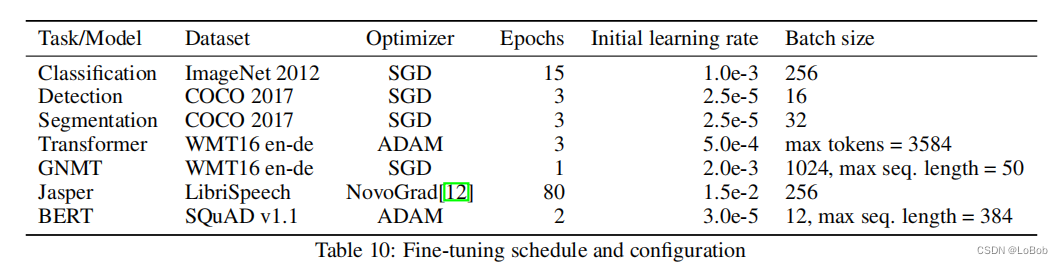# 深度学习Int8的部署推理原理和经验验证

## 深度学习Int8的部署推理原理和经验验证¶

#### 1、Introduction¶

1、32-bit的乘加变成了8-bit的乘加，同样的硬件单元下可以speed up；

2、32-bit的参数变成了8-bit，显然是model size变小了，需要的空间也变少了，ROM和RAM都减少了；

3、因为内存的要求降低了，对cache的利用率等其他内存系统操作等效率也会提高。

Table 1给出了理论位宽对吞吐、带宽等的要求，有用的信息是累加的数据类型，这一点在做量化部署实现的时候非常重要，其他数值只是个理论值，实际用处不大。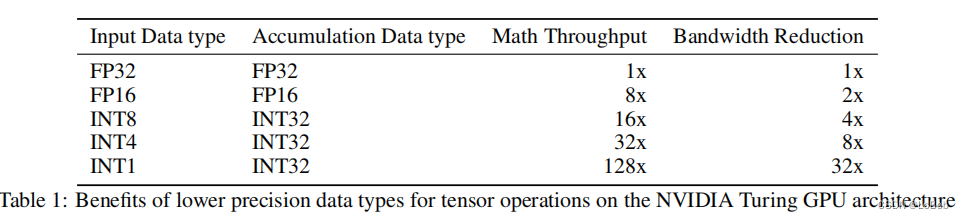#### 2、相关工作¶

1、《Quantization and training of neural networks for effificient integer-arithmetic-only inference》，也就是做量化必须看的paper，也是tflite的实现，也是目前QAT的做法的主流参考。这篇说的是如何用全整形进行推理，这部分我用c代码全部实现了(下次一定写稿)。

2、《Quantizing deep convolutional networks for effificient inference: A whitepaper》，白皮书，无需多言，必看paper，也是google出品。

3、《 Discovering low-precision networks close to full-precision networks for effificient embedded inference.》这篇说的是，在imagenet上，量化成int8后只需要1个epoch就会恢复到float-32的精度；一个trick即采用退火学习率调度和最后的学习率需要很小；截断阈值采用的百分比的策略。

4、《 Pact: Parameterized clipping activation for quantized neural networks.》，PACT讲的是激活函数的值域范围是可学习的。

#### 3、量化基础¶

2、将截断后的数值映射到整数上，这一步有round的操作。 如图所示：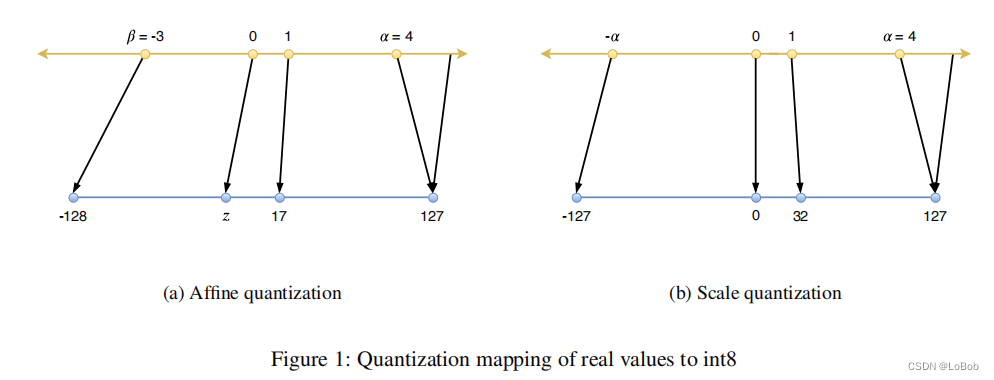Quantize：将一个实数转换为一个量化的整数， 即可float32 变成int8

Dequantize:将一个数从一个量化的整数表示形式转换为一个实数，即int8变成float32

Range Mapping：即一组数值的范围 [β, α]

Affifine Quantizationf(x) = s · x + z ，即非对称量化，s是缩放因子，z是零点，对于int8，那么int8的值域范围就是[0,255]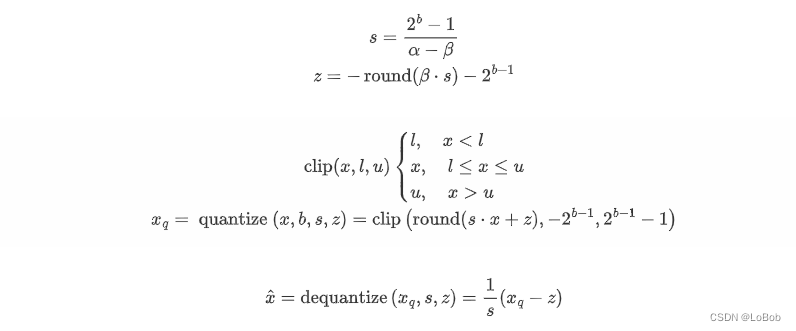Scale Quantizationf(x) = s · x, 即对称量化，对于int8，那么int8的值域范围就是[-127, 127]，不适用128这个数值，原因在IAQ论文说了是为了能用16-bit的累加器来存int8*int8，因为永远不存在-128 × -128，也就是改乘法的结果的绝对值不会超过2^14，可以保证用16-bit的累加器来存这个乘法结果。

\begin{gathered} s=\frac{2^{b-1}-1}{\alpha} \\ x_{q}=\operatorname{quantize}(x, b, s)=\operatorname{clip}\left(\operatorname{round}(s \cdot x),-2^{b-1}+1,2^{b-1}-1\right) \end{gathered}
\hat{x}=\operatorname{dequantize}\left(x_{q}, s\right)=\frac{1}{s} x_{q}

Tensor Quantization Granularity：per-tensor & per-channel: 这里更加细致地分析了颗粒度如何从per-tensor变到per-channel，还有中间心态的颗粒度，但实际并不会做太多骚操作，感兴趣的朋友们可以翻原文来了解。per-tensor就是整个神经网络层用一组量化参数(scale, zero-point)，per-channel就是一层神经网络每个通道用一组量化参数(scale, zero-point)。那么就是per-channel需要存更多的量化参数，对gemm的计算也有一点影响。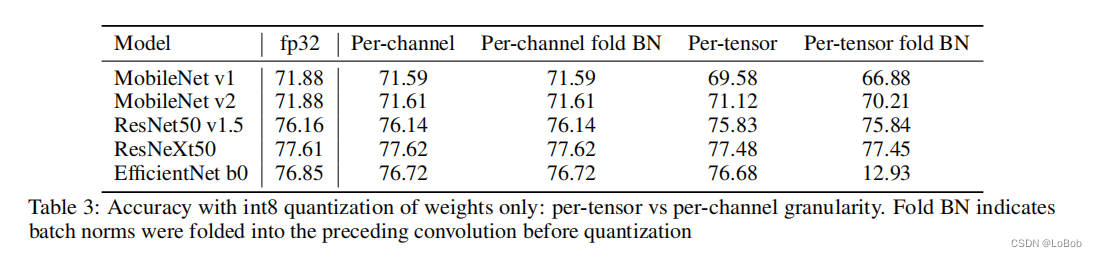Affifine Quantization即非对称量化在做矩阵乘法的时候比对称量化多了好几项：

\begin{aligned} y_{i j} & \approx \sum_{k=1}^{p} \frac{1}{s_{x}}\left(x_{q, i k}-z_{x}\right) \frac{1}{s_{w, j}}\left(w_{q, k j}-z_{w, j}\right) \\ &=\frac{1}{s_{x} s_{w, j}}\left(\sum_{k=1}^{p} x_{q, i k} w_{q, k j}-\sum_{k=1}^{p}\left(w_{q, k j} z_{x}+z_{x} z_{w, j}\right)-\sum_{k=1}^{p} x_{q, i k} z_{w, j}\right) \end{aligned}

\begin{aligned}y_{i j} & \approx \sum_{k=1}^{p} \frac{1}{s_{x}}\left(x_{q, i k}\right) \frac{1}{s_{w, j}}\left(w_{q, k j}\right) \\&=\frac{1}{s_{x} s_{w, j}}\left(\sum_{k=1}^{p} x_{q, i k} w_{q, k j}\right)\end{aligned}

\begin{aligned}\sum_{k=1}^{p}\left(w_{q, k j} z_{x}+z_{x} z_{w, j}\right)\end{aligned}

\begin{aligned}\sum_{k=1}^{p} x_{q, i k} z_{w, j})\end{aligned}

Calibration：Calibration是用来选模型参数和激活值的最大值和最小值，用来做截断。

max-min：tensor的最大值和最小值，这个策略没有截断。

KL散度（KL divergence）：最小化量化后int8与float32数据的KL散度，tensorrt采用这个策略。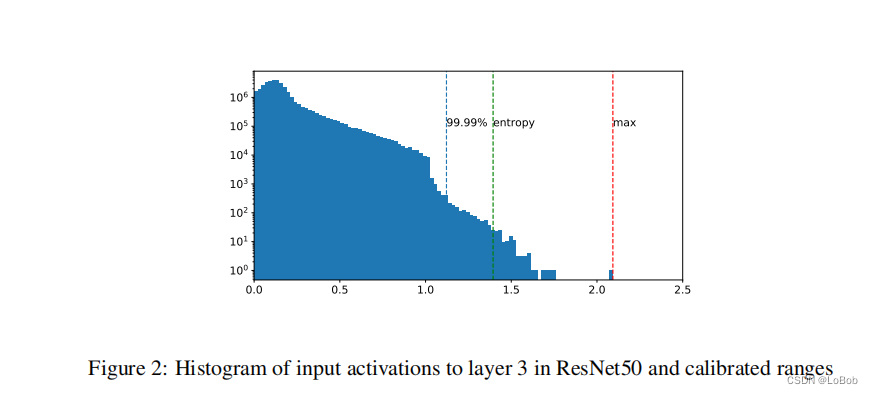#### 4、PTQ：训练后量化¶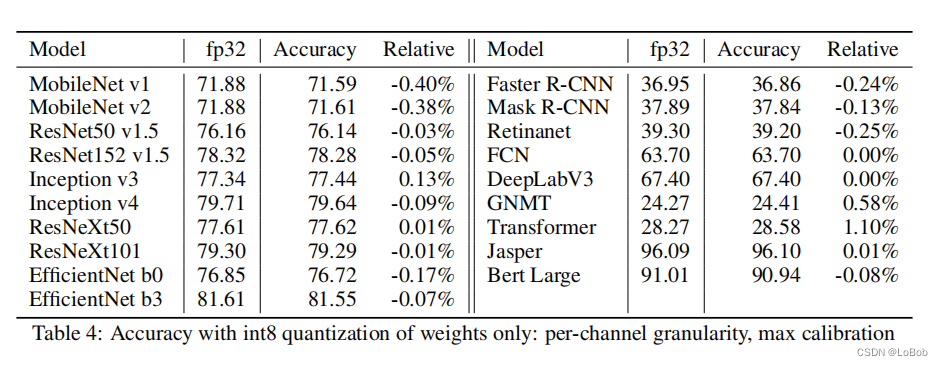tabel 4仅仅只是weight量化那肯定是不够的，需要对activation也量化做的量化才是实际部署使用的，效果如下图table 5，可以看到不用的模型/任务(分类/检测/NLP)，需要采用的校准策略是不一样的，也就是校准策略没有哪一中可以称霸。基本int8的量化，在分类、检测、NLP上精度损失都不大，是可以真实落地使用的量化比特位。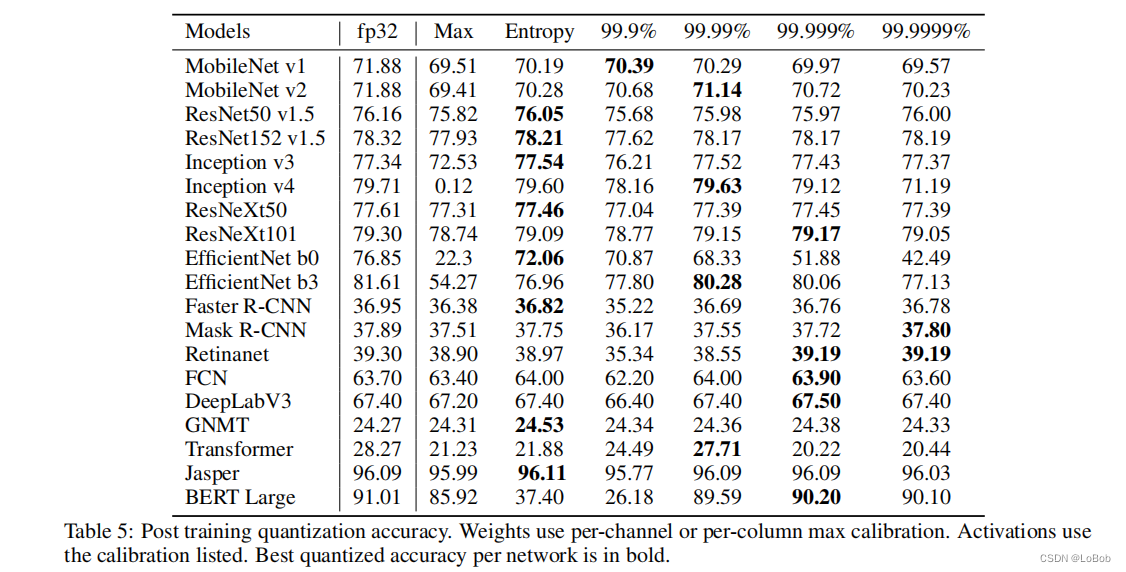#### 5、恢复精度的技术：Partial Quantization(部分量化)和QAT(量化中训练)¶

Partial Quantization：量化后对模型效果影响比较大（也就是更加敏感）的就用高比特来表示，用float32/float16/int16。这个敏感度判别采用最直接简单的方法：每次只量化一层跑一遍，看模型效果的影响，影响大的就更敏感，反之就不敏感。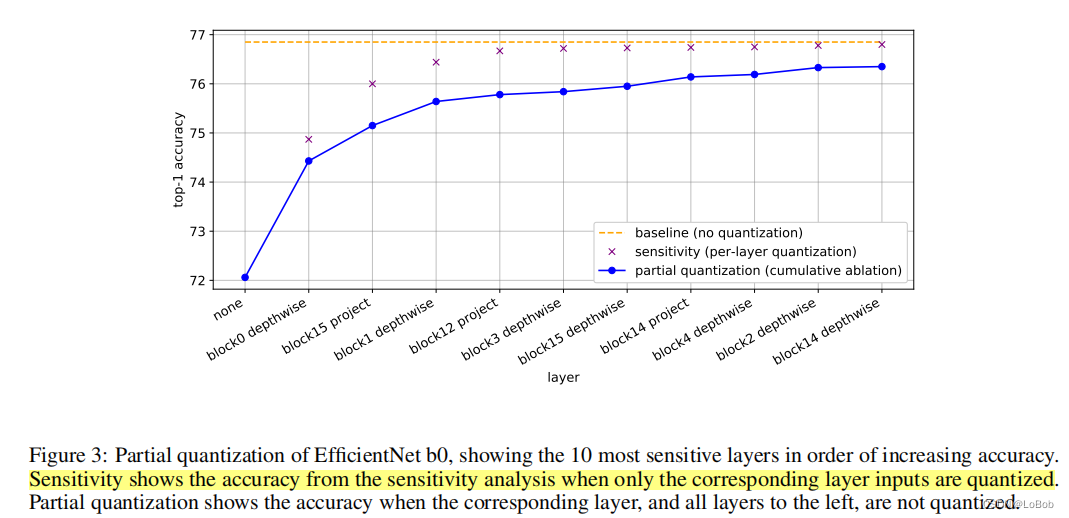Quantization-Aware Training(QAT): QAT就是在训练中插入fake quantize节点 做dequantize(quantize(x, b, s), b, s)，对quantize这个节点因为导数为0，采用STE(即梯度为0)来解决。QAT的实验如下table 7，会发现有些情况下QAT-int8比float32还好一丢丢，这时候论文作者的描述格局非常大：”Likewise, we do not interpret cases where accuracy is higher than fp32 as quantization acting as a regularizer, it is more likely to be noise or the result of the additional fifine-tuning.“，意思就是这只是实验中的“噪声”，也就是并不是方法多么sota，就只是实验的随机性罢了。大格局！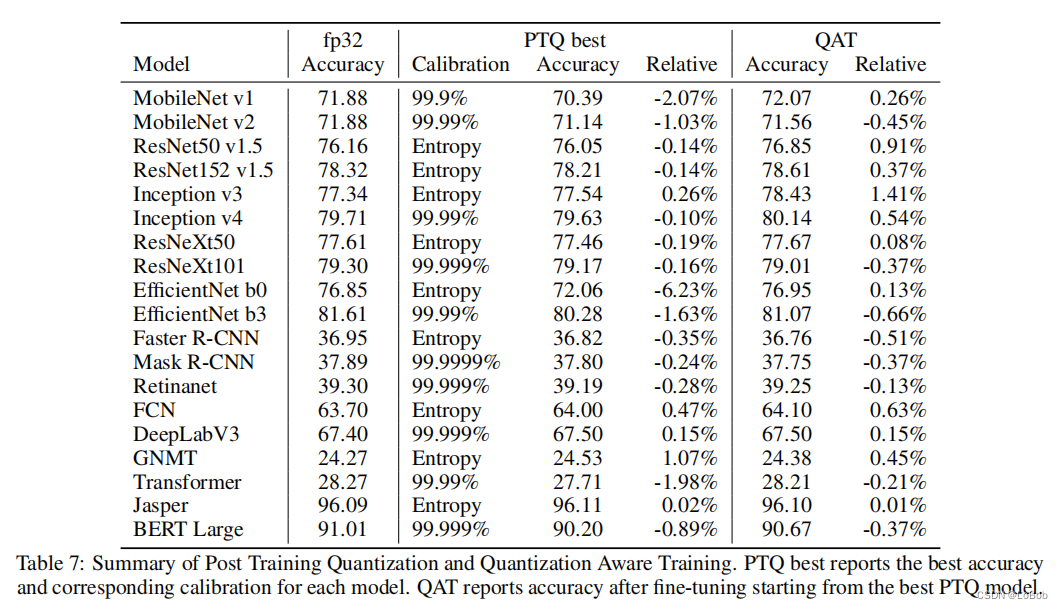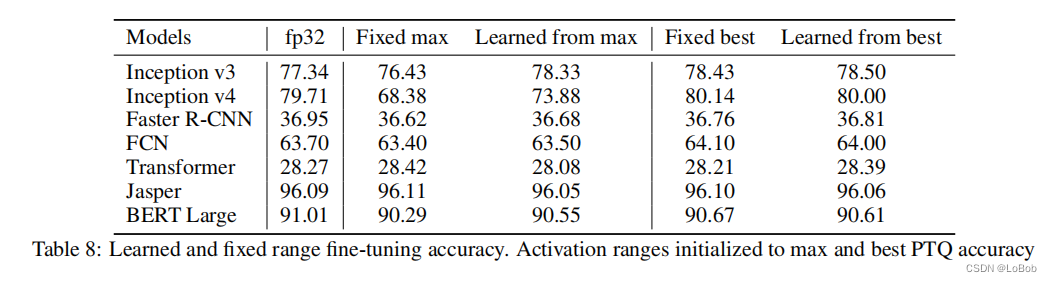#### 6. 推荐的量化的流程图¶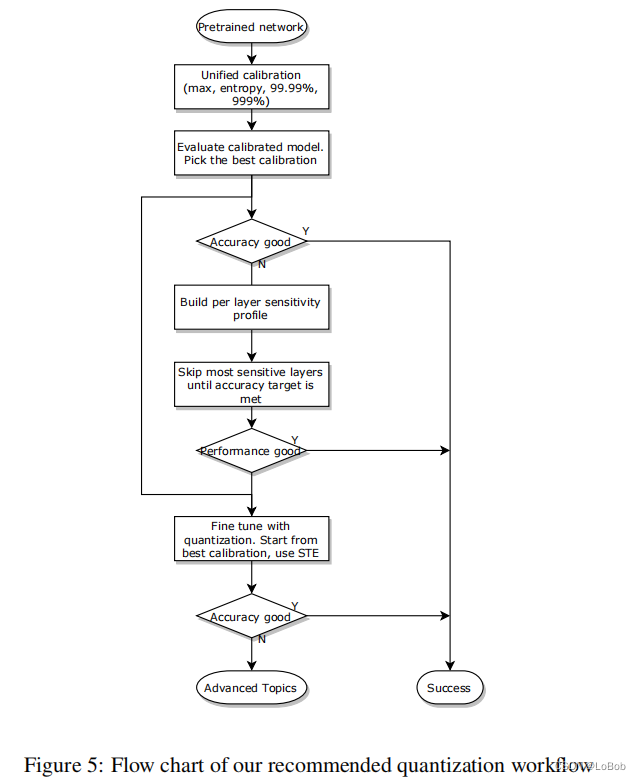weight：对称量化+per-channel+max-min校准策略+[-127, 127] activation：per-tensor+对称量化

Partial Quantization：只量化某一层跑推理，记录模型效果并且排序，用来选择敏感度，敏感度大的用高精度；，还是达不到要求用QAT；

QAT：加载效果最好的量化模型参数，插入伪量化节点，用原来初始学习率的1%做完QAT的初始学习率，采用退化学习率衰减器。